# Evaluation

## Comparison of CFD calculations with Experiments

Results for the two flow conditions reported in Table 1 are discussed in the book of the ECARP project  and are summarized hereafter.

Flow condition 1 (Mach=0.17, Reynolds=7.7x106, α=10° , transition fixed)

A comparison between the computed and the measured (from DLR NWG wind tunnel) pressure coefficients at several cross sections is presented in the figures 3, and 4.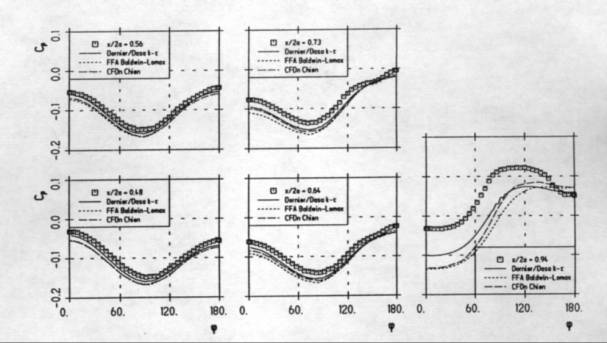Figure 3 : Flow condition 1 : Pressure coefficient at various cross sections - results by Dornier/DASA-LM, CFD-Norway and FFA (page 457 of the ECARP book )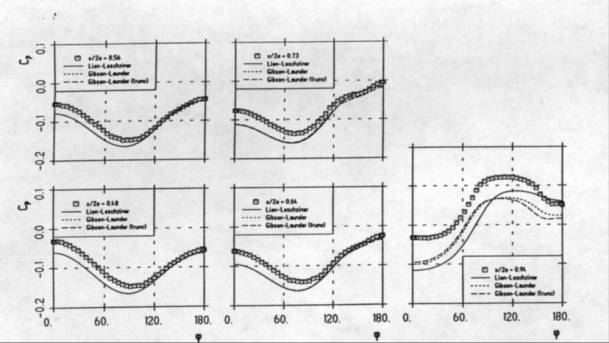Figure 4 : Flow condition 1 : Pressure coefficient at various cross sections - results by UMIST

(page 458 of the ECARP book )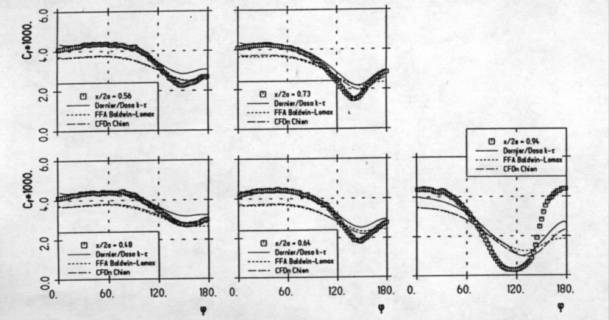Figure 5 : Flow condition 1 : Skin friction coefficients at various cross sections - results by Dornier/DASA-LM, CFD-Norway and FFA (page 458 of the ECARP book )

As pointed out in the ECARP book , the flow, by a comparison of the Navier-Stokes results with the potential theory (Meier et al. 1986 ), remains attached up to the station at x/2a=0.73. In this region, the numerical results present an acceptable agreement with the experimental data, while differences between computed and measurements data appear in the region of separated flow. The constant under-prediction of the pressure distributions is likely due to an un-correct measurement of the reference pressure.

The skin friction coefficients, defined as the total wall shear stress divided by the free-stream dynamic pressure, are shown in the figures 5 and 6. The agreement with the experimental data is acceptable in the attached flow region, while large discrepancies can be noticed in the rear part of the spheroid where the flow is separated.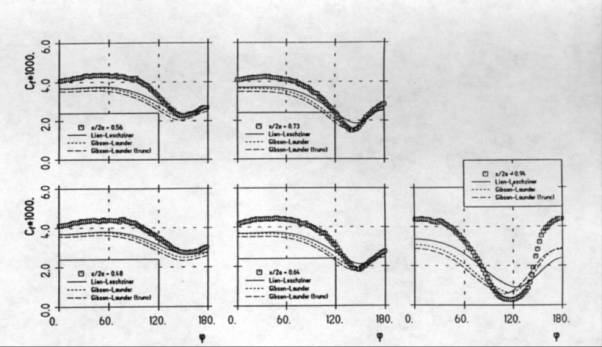Figure 6 : Flow condition 1 : Skin friction coefficients at various cross sections - results by UMIST

(page 459 of the ECARP book )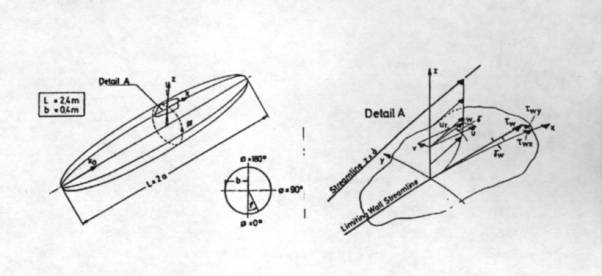Figure 7 : Coordinate system for the spheroid (page 459 of the ECARP book )

The figures 8 and 9 present the wall shear stress angle defined as the deviation of the friction line from the constant azimuth angle on the spheroid surface (see figure 7 for the coordinate system used).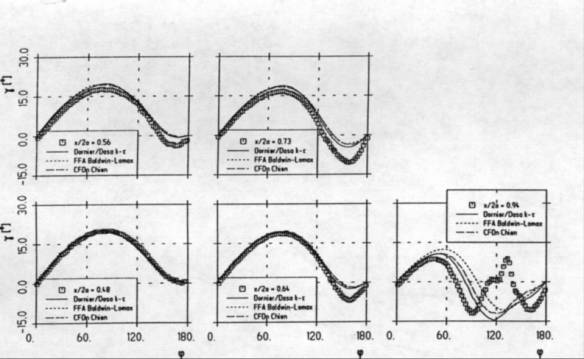Figure 8 : Flow condition 1 : Wall shear stress angle - results by Dornier/DASA-LM, CFD-Norway and FFA

(page 460 of the ECARP book )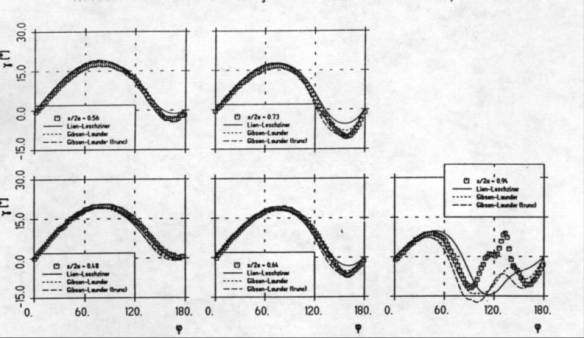Figure 9 : Flow condition 1 : Wall shear stress angle - results by UMIST(page 460 of the ECARP book )

The comparison with the measurements is globally good, and the shortcomings of the turbulence models in the region of separated flow are clearly visible. At the last station, at x/2a=0.94, only the Reynolds stress models by UMIST are able to reproduce, at least qualitatively, the experimental results.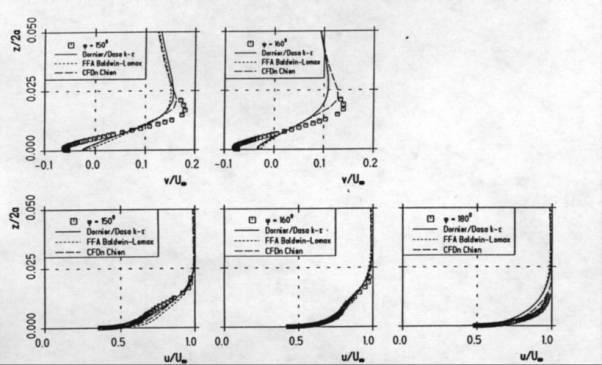Figure 10 : Flow condition 1 :Velocity profiles at x/2a=0.73 - results by Dornier/DASA-LM, CFD-Norway and FFA (page 462 of the ECARP book )

Stream-wise and azimuthal velocity components at x/2a=0.73 at several circumferential angles are presented in the figures 10 and 11 . At this station the flow is approaching the separation but is still attached and all the turbulence models, included the Baldwin-Lomax, provide good results. A better agreement with the experimental data for the azimuthal velocity component is provided by the Reynolds stress models used by UMIST.

The results achieved by UMIST are discussed in more detail in Lien and Leschziner (1996) . The importance of removing a fragment of the wall-related pressure—strain correction term in the Gibson and Launder Reynolds stress model  adopted in the simulations is recognized.

Flow condition 2 (Mach=0.25, Reynolds=6.5x106, α=30° , transition free)

This was an optional flow condition in the ECARP project, and only results by CFD-Norway and UMIST are available.

The pressure coefficients are shown in figure 12. A good agreement between numerical and experimental results can be noticed on the windward side of the spheroid with the shift in the reference pressure, due to pressure variations larger than for flow condition 1, not playing a significant role. The minimum of the pressure coefficient is under-predicted by the linear κ-ε models and all the turbulence models detect a flow separation on the leeward side of the body. The differences present at x/2a=0.94 could be due to influence of the transition not taken into account in the computations.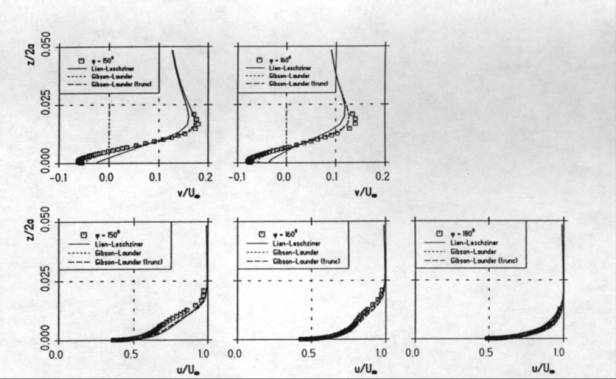Figure 11 : Flow condition 1 :Velocity profiles at x/2a=0.73 - results by UMIST

(page 463 of the ECARP book )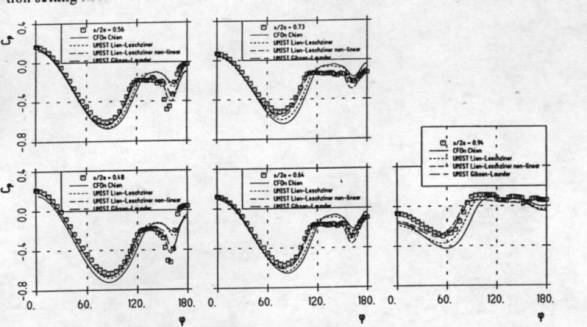Figure 12 : Flow condition 2 : Pressure coefficients at various cross sections (page 464 of the ECARP book )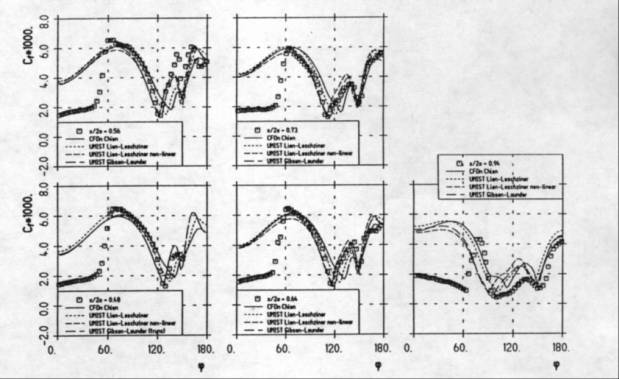Figure 13 : Flow condition 2 : Skin friction coefficients at various cross sections

(page 465 of the ECARP book )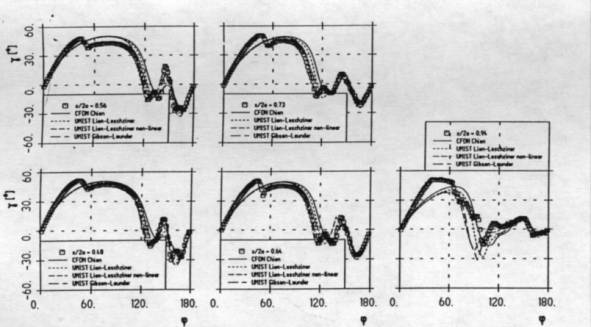Figure 14 : Flow condition 2 : Wall shear stress angle - (page 466 of the ECARP book )

The friction coefficients are shown in figure 13. A large discrepancy can be seen in the laminar region because the numerical simulations were performed by assuming fully turbulent conditions. However, the experimental levels of the skin friction coefficients are recovered in the turbulent region of the flow.

Results for the shear stress angles are shown in figure 14. All the numerical results present the same number of deflection points and the same vortex structure. The measured and numerical stream-lines, as computed by the Gibson and Launder RSTM (top left plot), linear Lien and Leschziner κ-ε (top right plot), Chien κ-ε (bottom left plot), and non linear Lien and Leschziner κ-ε (bottom right plot), are shown in figure 15 and 16 respectively. The extension of the separated flow region in the leeward upper side is over-estimated especially towards the rear end of the body. The experimental flow field (figure 15) suggests the existence of three separation lines. The intermediate separation line at about φ=120° , as could be also seen in figure 14 where γ=0 means either separation or reattachment, seems to have not been captured by the numerical simulations.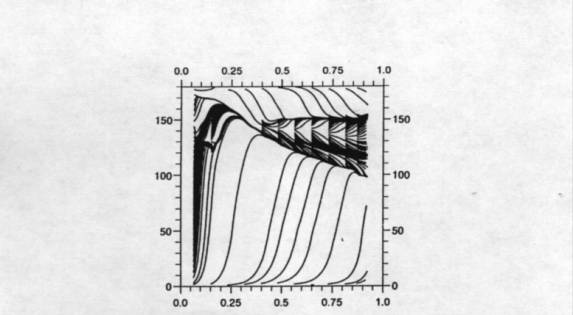Figure 15 : Flow condition 2 : Experimental surface stream-lines (page 466 of the ECARP book )

The non linear κ-ε and the Reynolds stress turbulence models provide a better prediction of the separated zone. This is also confirmed by the computed pressure coefficient (figure 12), where, mainly at the last station, the linear κ-ε models under-estimate the extension of the separated region and over-estimate the pressure recovery .

The flow around a spheroid with the flow specification 2 has been simulated by Lien (1996)  by using a 653 and 1283 nodes grids. The linear, quadratic and cubic version of the turbulence model by Lien et al. (1995)  have been used. The best results have been provided by the cubic model due to its ability to account for the interaction between streamline curvature and turbulence.

This flow condition has been simulated by Lien and Durbin (1996)  on a 653 grid using the low Reynolds κ-ε model by Lien and Leschziner (1993)  and a κ-ε-v2 model  with the Launder and Kato’s modification for the production of the turbulent kinetic energy . This modification has the aim to avoid the defect shown by any conventional linear turbulence model to generate too high level of turbulence energy in the stagnation regions and is similar to the realizability constraints for the turbulence time scale suggested by Durbin . From the results shown in  it is possible to see that at x/2a =0.223 the κ-ε-v2 model with the Launder and Kato’s modification, although unable to predict a natural transition, presents a transition like behaviour . The extension of the separated zone is under-estimated by both the models with slightly better results provided by the κ-ε-v2 . However, some of the discrepancies between numerical and experimental data might be partially attributed to the grid density too coarse especially close to the rear end of the spheroid.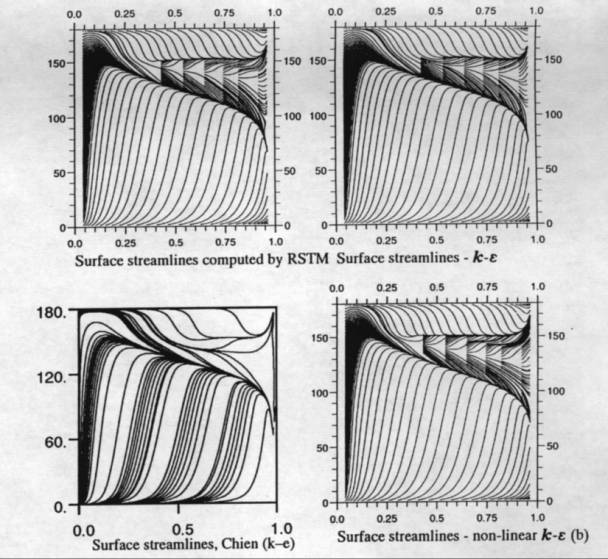Figure 16 : Flow condition 2 : Computed surface stream-lines (page 467 of the ECARP book )